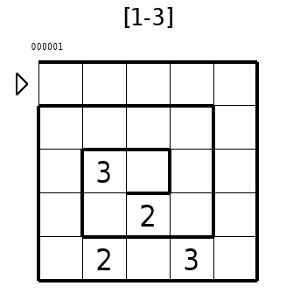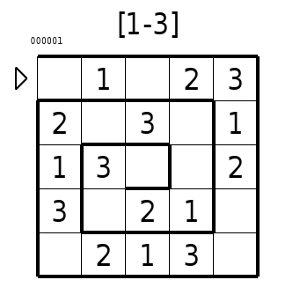Rules of Sudoku Labyrinth

## Rules of Sudoku Labyrinth

The Sudoku Labyrinth (sometime named: Snail or Magic Spiral) is a very addictive puzzle.

Each grid contains a path that visits each cell of the grid. The beginning of the path is indicated by an arrow.
The goal of the puzzle is to repeat the number sequence “1-2-3” in the path as many times as possible until the end of that path; each row and column have all the numbers of the sequence only once.Enter the sequence, following the direction of the path, from the beginning to the end. Each number of sequence must be placed in increasing order: (1-2-3-1-2-3-..1-2-3).
The path could be in the form of a spiral, like a snail (this is why this puzzle is called magic spiral), or in the form of a labyrinth. The number sequence is generally 1-2-3, but for  larger grid it could be 1 to 4. The size of the sequence is indicated at the bottom of each puzzle by [1-3] or [1-4].
In this puzzle, like Sudoku, some clues are given. You only need to use logic to resolve this puzzle; each grid has only one solution.
The rules are:

1. Each row and column must contain each number of the sequence only once.
2. The sequences are repeated (in increasing order) in the direction of the path.
3. Each sequence must be complete (containing all the number).

Rule 3 implies that the last number in the path must be the last number of the sequence, otherwise the last sequence would be incomplete.
But, enough discussion, let’s see how the above applies to the resolution of a grid with the sequence 1-2-3.

This is a magic spiral with the entrance is at the top left. Each sequence (1-2-3) must be entered, starting at this entrance, one after another.
Note in the first row of this grid, a 2 at D1 and a 3 at E1. According to rule 1, the row must have all the numbers only once, so the 1 is missing. The number 1 could be in one of the three empty cells (A1,B1,C1). But, in column C, a 1 is present (in C5), then 1 can’t be put in cell C1 (since the third column would have two 1’s – in violation of rule 1). The 1 must go in either A1 or B1; for now, we don’t know exactly which one, but, in order to help us resolve the grid, we could mark cell C1 with an X to indicate an empty cell.
The third column (C) has a 1 and a 3, then 2 is missing, and two cells are free C3 and C4 (remember we have eliminated C1) . The cell C3 is the last cell of the path, then if this cell is not empty, then the cell must have the last number of the sequence ( a 3 following rule 3) so a 2 cannot be there because there would be no room after it to put the 3. The only available cell in the third column is C4, so we put the 2 in C4 and mark cell C3 with an X .

We have progressed. Now, in following the path we note that the last column (E) begin with 3 (E1), then we have to follow with 1 and 2, in that order, in this column. The last two cells (E4 and E5) cannot accept a 2 because those rows already have a 2 (row 4 and row 5), leaving only cells E2 and E3. Cell E2 immediately follows a 3 so this cell, if it is occupied, can only accept a 1, otherwise the sequence would be broken, so cell E3 will have the 2 and, by the way, E2 has the 1. We can also mark the cells E4 and E5 as empty.

Now we note that the last number of the last column (E) is a 2, then the next number must be 3 and the only free cell in the path (the spiral) is D5. Then a 3 goes in D5; this completes the last row and we can mark the cell A5 as empty. Following the path, we notice that cell B2 could only accept a 2 (because this cell is immediately followed by a 3 in row 2), but column B already has a 2; since it can’t accept a 2, then cell B2 must be empty (and gets a mark).

We only have three free cells in the path (spiral) between the number 2 (B5) and the number 3 (C2). If all three of these cells were empty, then rule 2 would not be broken, but the first column would have only one cell left (A1); then column A would not have all the numbers (rule 1). So, the three free cells must be occupied by a complete sequence (3,1 and 2 respectively in A4,A3 and A2). As the first column (A) is complete, we mark cell A1 with an X (empty). Now cell B1 is the only place to accept the 1 in the first row (1).

We have made a lot of progress, we must put the 3 in the second column (B) and the 1 in the fourth (D) column. The only cell in column B that can accept a 3 is B3 and, by the way, the 1 goes in D4 for the solution.